2. Dialectical Judgements and the Quantitative-Qualitative Numerical Field

L. G. Kreidik (translation from Russian T. S. Kortneva and G. P. Shpenkov)

2.1. Relation between elements of oppositi and their measures

From point of view contradictoriness and noncontradictoriness, all objects of thought and related to them objects of nature in the first approximation can decompose on the four kinds:

Objects of thought, facets of subjects and their sides are evaluated by

quantitative, qualitative and quantitative-qualitative measures.

Relations between elements of oppositi are characterized by their measures which in a general case depend on the character of the relations, elements of the oppositus, and the environmental parameters. The measure of the relation is expressed by the equality: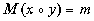(1.2)

where the left-hand side is the name of the measure of the relation between the elements x and , the right-hand side is the value of the measure.

In a general case, oppositi are multielements, then the measure of relations between them is expressed by the formula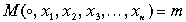. (1.3)

If the relation and the measure are fixed, then there is a definite quantitative relation between measures of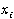. Let the first k componentsbe able to assume arbitrary values within some admissible ranges and the values of the other n � k measures of. depend on them. We will introduce the multielement oppositus of independent elements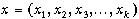and the multielement oppositus of dependent arguments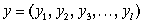,

where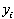denotes dependent measures .; and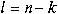.

The measure of the relation between the elements of oppositi are expressed by (1.2). If m = y, then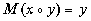(1.4)

In this case x and are related by the relation denoted by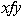or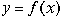and called a function. In a general case, the measures of x and are quantitative-qualitative. Thus, the function is defined as the relation between the measures of x and with the measure y. Extending formula (1.4) to functions, it is possible to speak about the relation L between the functions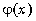and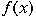with the measure P: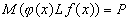(1.5)

In a general case arguments of the functions can be different: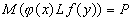. (1.5a)

If P is a number or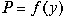, the relation is expressed by a functional or an operator, respectively: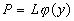. (1.6)

Any object of nature is inseparable from the set of relations relating it to the enclosing World. Interrelation of an object of nature x and its relation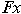with

the environment is described by the opposite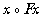, in which the relation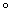is the relation between the object x and the relation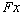.

If two objects X and with the relationsand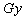are interrelated by the relation with the measure P, we have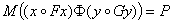(1.7)

Depending on the type of the measure P, we can have various formations. When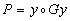, the relation is called a functor, here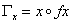, where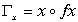and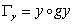. (1.8)

If P is a number, it seems reasonable to call the relation a functoral functional. At present it has become necessary to introduce such notions into physics since they play an important role in it, although they are not defined explicitly.

In a simplest case the measure of the relation is a function of the relation itself and the measures of the elements a and b: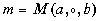or briefly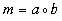. (1.9)

In general, the measure of a relation also depends on environmental parameters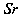: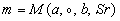. (1.10)

Here, the environment appears as a superstructure over the structure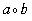.

The procedure used to determine the measure of relations is called a logical operation or simply an operation. The general name of the operation will be denoted by " *". In a simplest case, the function of the measure of relations is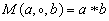. (1.11)

Then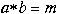. (1.12)

In such a situation the left-hand side of equality (1.11) can be expressed, naturally, in a brief form: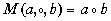. (1.13)

Following this form, we have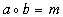. (1.14)

Equalities (1.12) and (1.14) allow us to write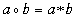. (1.15)

Here the relation " � " and the operation "*", different in contents, are identified by form. In this situation, dialectic says: and unequal are equal. This situation is typical for science. The relation and operation, such as quality and quantity, are polar-opposite: the relation is negation of the operation: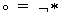.

Fundamentals relations are represented by polar-opposite relations, namely, additive and multiplicative relations, with their oppositi: summation and subtraction, multiplication and division. There are operations with the same names and measures, corresponding to them: the sum and difference, the product and quotient.

2.2. Quantitative and qualitative elements of a judgement

As has been already mentioned, a simple description of an object of thought usually contains the statement Q and judgement Z. Both these notions constitute a complex formation called a reasoning Ra about the object of thought. To some extent, the statement is a qualitative component of the reasoning and the judgement is a quantitative component of its. The reasoning Ra is expressed by the matrix, a logical vector,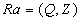.

If Q and Z are pure quality and quantity, then the reasoning is expressed by antilogies. In a general case, Q and Z are complex contradictory components of the reasoning vector with predominance of quality in Q and quantity, in Z . This is characteristic of objects of thought as subjective images of objective subjects of nature.

We will consider simplest meanings of judgements. If Z is an elementary dialectical affirmative judgement, its possible meanings are: si (yes ), a neutral affirmation or briefly, an affirmation; +si (+yes), a positive affirmation; and �si (- yes) , a negative affirmation. Here are some examples of such kind of judgements:

a) si = " a body moves with the velocity si between the points A and B";

b) +si = "a body moves with the velocity si from the point A to the point B";

c) �si = "a body moves with the velocity si from the point to the point A".

If Z is an elementary dialectical negative judgement, its possible meanings are:

no, a neutral negation or briefly, a negation;

+no, a positive negation;

- no, a negative negation.

Below are some examples of them:

a) no = "a body does not move with a velocity no between the points A and B";

b) +no = "a body does not move with a velocity no from the point A to the point B";

c) - no = "a body does not move with a velocity no from the point to the point A".

Additive oppositi-judgements or combined judgements Z assume binary meanings: a) si+yes, affirmation-affirmation; b) si+no, affirmation-negation; c) no+si, negation-affirmation; d) no+not, negation-negation. Here and further, si, yes and no, not are judgements of the same kind but the different measures. They reflect noncontradictions, oppositions, and contradictions of the object of thought.

Multiplicative oppositi-judgements or systems judgements Z have meanings: a) si-yes, affirmation of affirmation; b) no-yes, affirmation of negation; c) si-no, negation of affirmation; d) no-not, negation of negation.

Affirmations +si, -si (negations +no, -no) are related to affirmation si (negation no) as particular and general. Therefore, in a general case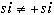(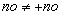) and especially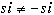(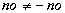).

2.3. Measures of judgements

If it is necessary to emphasize, neutral elementary judgements Z should be placed between two vertical lines,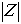. The symbol "+" of positive judgements will be omitted in logical expressions which do not contain the general judgements. In particular, a positive judgement of +kind is wrote by the neutral form.

Elementary judgements of affirmation with a unit measure will be named unit affirmations and denoted by e or 1. The number a of the units will be assumed to be a measure of the affirmative judgement: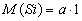or briefly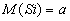,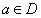. (1.16)

The affirmation is positively if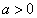, otherwise it is negative.

Elementary judgements of a polar negation (see sect. 1.1.4) with a unit measure will be named unit polar negations and denoted by i,j, etc. The number b of the units will be assumed to be a measure of the polar negation judgement: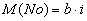or briefly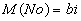,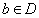. (1.17)

The negation is positively if b>0, otherwise it is negative.

Judgements with measures a and bi are polar-opposite judgements-antilogies, whereas judgements with measures a and b (correspondingly ai and bi) are simply opposite judgements-antonyms, if a and b are opposite by sign.

Two judgements X and are equioppositi, if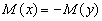. (1.18)

Additive and multiplicative contradictory judgements with polar opposed semi-opposites will be expressed as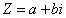,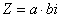or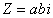. (1.19)

Let us agree an a-semi-oppositus call the material component of the oppositus (1.19), meaning the word "material" as a general name of a-semi-oppositi. In very specific case, the adjective "material" can assume the more concrete sense. Correspondingly, the polar opposed bi-semi-oppositus will be called the ideal component of the opposite (1.19), meaning the word "ideal" as a general name of Zn-semi-oppositi.

Binomials (1.19), as contradictory measures of oppositi, will be called moduses of oppositi, or complex modules, and denoted by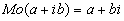and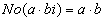. (1.20)

An oppositus is also characterized by a norm denoted by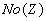, additive and multiplicative norms are defined by the equalities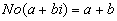and. (1.21)

Important measures are quantitative modules of additive and multiplicative oppositi given by the formulae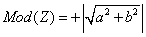,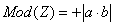. (1.22)

The modules will be briefly expressed by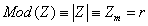, etc. (1.23)

The quantity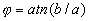(1.24)

is a phase of the oppositus.

In terms of quantitative phase of the oppositus, any oppositus with an arbitrary relation between its elements can be expressed in a compact form, describing not only measures of the elements of the opposite but also quantitative relations between them, for example: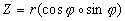. (1.25)

Measures of elements of the oppositus and elements themselves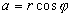and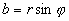. (1.26)

can be a scalar and a vector and the phase of the oppositus is not related with their directions. The modulus and phase of the oppositus is the modulus and phase of a dialectical judgement changing following changes in the object of thought. The notion "an elementary contradictory vector-oppositus" will be related to the product of the scalar measure of the oppositus z by unit vector of the direction n:. (1.27)

The longitudinal scalar product of two vectors is the scalar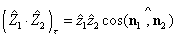. (1.28)

If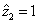, the scalar product (1.28) defines the longitudinal projection of the vector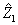, into the vector direction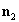.

The transverse scalar product of two vectors is the scalar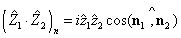. (1.29)

where i is the unit of polar negation.

If, the scalar product (1.29) defines the transverse projection of thevector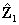, into the vector direction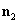. The transverse scalar product is negation of the longitudinal scalar product. The scalar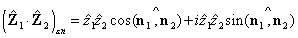(1.30)

is called a longitudinal-transverse scalar product of two vectors.

Finally, the total scalar product of two vectors will be called the total scalar product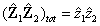(1.30a)

The sum of elementary vectors-oppositi gives a composite vector-oppositus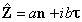(1.31)

where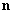and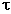are the vectors of two arbitrary directions.

The more important case of the complex vector is that one with the mutually perpendicular vectorsand.

The scalar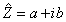(1.31a)

will be called a modus, or a complex modulus, of the vector (1.31).

If measures a and b are contradictorily, the vector-oppositus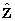has the form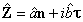(1.31b)

with the complex modulus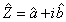. (1.31c)

The complex modulus (1.31c) of the vector-oppositus (1.31b) is the measure possessing features both a vector and scalar.

A vector-oppositus and its modus will be usually designated, for simplicity, by the same symbol. If it is necessary to emphasize the vector-oppositus, its symbol will be wrote by bold.

2.4. A space graph of a dialectical judgement

A variable oppositus-judgement suitably to depict graphically in the space of objective-subjective reality in the form of two mutually perpendicular graphs of changes in the elements of the judgement (Fig. 1.5). The plane of the graph of the dialectical judgement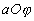is the plane of affirmation and the plane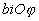is the plane of negation. In a general case, the planes of affirmation and negation will be called material and ideal planes, respectively. Intersection of the affirmation and negation planes passes along the axis of the phase of the judgement (along the beam of the judgement). Intersection of the judgement by the frontal plane F determines the instan-taneous value of the judgement S=x+iy. Therefore, F is a plane of the present for a particular value of the phase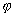. It separates the past and future states of the object of thought.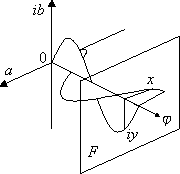Fig. 1.5. A three-dimensional graph of a judgement.

For convenience, contradictory aspects of a judgement can be expressed occasionally in a plane (Fig. 1.6).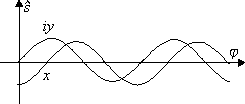Fig. 1.6. A two-dimentional graph of a dialectical judgement.

Abstract graphs of judgements-measures of subjective dialectics can coincide with those of objective dialectics completely or partially. In a general case, measures of the components of a dialectical judgement are a complicated complex of the basis and superstructure: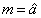, (1.32)

where a is a basis, the core of the measure, a quantitative feature of the value.

The sign "^" above the core symbolizes here the superstructure (envelope of the measure), which is expressed by a set of various sings that refer to the basis.

In dialectics, an estimation of the truth and falsity of oppositi, describing objects of thought, is realized by the oppositi whose components of affirmation and negation express the measures of truth and falsity, respectively. These logical judgements about judgements will be called the oppositi-opinions or simpler, opinions (from the Latin, opinio-view).

Evidently, opinions themselves can be analyzed from point of view their truth-falsity. Therefore, it makes sense to consider also logical judgements about the judgements-opinions. Such judgements will be called oppositi-gnomes (from the Greek, gnomh = opinion) or simpler gnomes. Opinions and gnomes are peculiar the first and second logical derivatives of oppositi-judgements, describing objects of thought. In real life, the judgements about fairness of some affirmations, opinions, and theories are not restricted solely by the logical derivatives of the second orders.

Theoretical Dialectical Journal: Physics-Mathematics-Logic-Philosophy, N.1, site http://www.tedial.narod.ru/